1593894120

# Master Pandas’ Groupby for Efficient Data Summarizing And Analysis

## Learn to group the data and summarize in several different ways, to use aggregate functions, data transformation, filter, map, apply a function in the DataFrame, and visualization using groupby.Source: Unspalsh by Ilona Froehlich

Groupby is a very popular function in Pandas. This is very good at summarising, transforming, filtering, and a few other very essential data analysis tasks. In this article, I will explain the application of groupby function in detail with example.

## rashida048/Datasets

Contribute to rashida048/Datasets development by creating an account on GitHub.

github.com

Here I am importing the necessary packages and the dataset:

``````import pandas as pd
import numpy as np
``````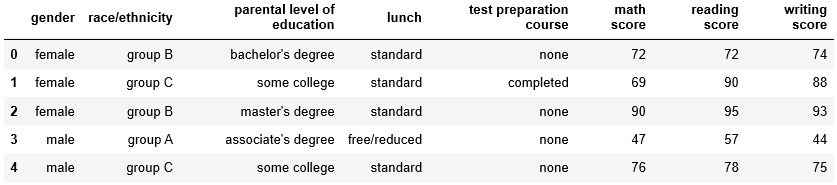## How Groupby Works?

Groupby function splits the dataset based on criteria that you define. Here I am showing the process behind the groupby function. It will give you an idea, how much work we may have to do if we would not have groupby function. I will make a new smaller dataset of two columns only to demonstrate in this section. The columns are ‘gender’ and ‘reading score’.

``````test = df[['gender', 'reading score']]
``````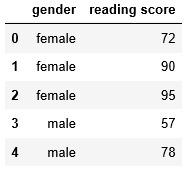Let’s find out the average reading score gender-wise

First, we need to split the dataset based on gender. Generate the data for females only.

``````female = test['gender'] == 'female'
``````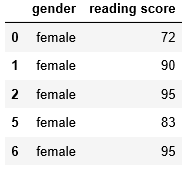In the same way, generate the data for the males,

``````male = test['gender'] == 'male'
``````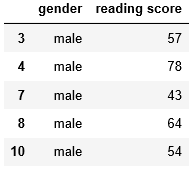Using females and males dataset above to calculate the mean reading score for females and males respectively.

``````fe_avg = test[female]['reading score'].mean()
print(fe_avg, male_avg)
``````

The mean reading score of females is 72.608 and the mean reading score for males is 65.473. Now, make a DataFrame for the mean reading score of females and males.

``````df_reading = pd.DataFrame({'Gender': ['female', 'male'], 'reading score': [fe_avg, male_avg]})
``````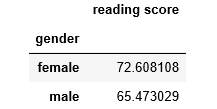Now, let’s solve the same problem with the groupby function. Splitting the data based on gender and applying the ‘mean’ on it with just one simple line of code:

``````test.groupby('gender').mean()
``````

This small piece of code gives the same result.

## Groups in Groupby

I will use the original dataset ‘df’ now. Make groups of ‘race/ethnicity’.

``````race = df.groupby('race/ethnicity')
print(race)
``````

Output: <pandas.core.groupby.generic.DataFrameGroupBy object at 0x0000023339DCE940>

It returns an object. Now check the datatype of ‘race’.

``````type(race)
``````

Output: pandas.core.groupby.generic.DataFrameGroupBy

So, we generated a DataFrameGroupBy object. Calling groups on this DataFrameGroupBy object will return the indices of each group.

``````race.groups

#Here is the Output:
{'group A': Int64Index([  3,  13,  14,  25,  46,  61,  62,  72,  77,  82,  88, 112, 129,              143, 150, 151, 170, 228, 250, 296, 300, 305, 327, 356, 365, 368,              378, 379, 384, 395, 401, 402, 423, 428, 433, 442, 444, 464, 467,              468, 483, 489, 490, 506, 511, 539, 546, 571, 575, 576, 586, 589,              591, 597, 614, 623, 635, 651, 653, 688, 697, 702, 705, 731, 741,              769, 778, 805, 810, 811, 816, 820, 830, 832, 837, 851, 892, 902,              911, 936, 943, 960, 966, 972, 974, 983, 985, 988, 994],             dtype='int64'),  'group B': Int64Index([  0,   2,   5,   6,   7,   9,  12,  17,  21,  26,              ...              919, 923, 944, 946, 948, 969, 976, 980, 982, 991],             dtype='int64', length=190),  'group C': Int64Index([  1,   4,  10,  15,  16,  18,  19,  23,  27,  28,              ...              963, 967, 971, 975, 977, 979, 984, 986, 996, 997],             dtype='int64', length=319),  'group D': Int64Index([  8,  11,  20,  22,  24,  29,  30,  33,  36,  37,              ...              965, 970, 973, 978, 981, 989, 992, 993, 998, 999],             dtype='int64', length=262),  'group E': Int64Index([ 32,  34,  35,  44,  50,  51,  56,  60,  76,  79,              ...              937, 949, 950, 952, 955, 962, 968, 987, 990, 995],             dtype='int64', length=140)}
``````

Have a look at the output above. Groupby function splits the data into subgroups and you can now see the indices of each subgroup. That’s great! But only the indices are not enough. We need to see the real data of each group. The function ‘get_group’ helps with that.

``````race.get_group('group B')
``````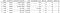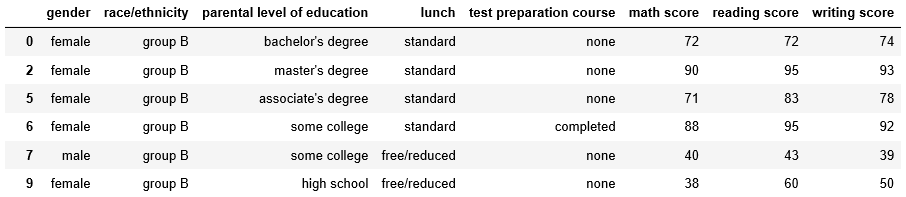I am showing the part of the results here. The original output is much bigger.

Find the size of each group

Calling size on the ‘race’ object will give the size of each group

``````race.size()
``````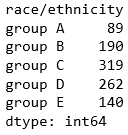Loop over each group

You can loop over the groups. Here is an example:

``````for name, group in race:
print(name, 'has', group.shape, 'data')
``````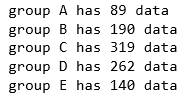Grouping by multiple variables

In all the examples above, we only grouped by one variable. But grouping by multiple variables is also possible. Here I am grouping by ‘race/ethnicity’ and ‘gender’. This should return the number of data of each race segregated by gender.

``````df.groupby(['gender', 'race/ethnicity']).size()
``````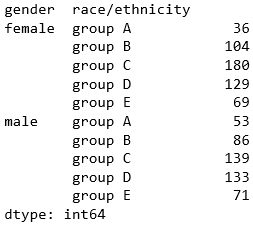This example aggregates the data using ‘size’. There are other aggregate functions as well. Here is the list of all the aggregate functions:

sum()

mean()

size()

count()

std()

var()

sem()

min()

median()

Please try them out. Just replace any of these aggregate functions instead of the ‘size’ in the above example.

Using multiple aggregate functions

The way we can use groupby on multiple variables, using multiple aggregate functions is also possible. This next example will group by ‘race/ethnicity and will aggregate using ‘max’ and ‘min’ functions.

#data-science #pandas #data-analysis #towards-data-science #python #data analysis

## Buddha Community1593894120

## Learn to group the data and summarize in several different ways, to use aggregate functions, data transformation, filter, map, apply a function in the DataFrame, and visualization using groupby.Source: Unspalsh by Ilona Froehlich

Groupby is a very popular function in Pandas. This is very good at summarising, transforming, filtering, and a few other very essential data analysis tasks. In this article, I will explain the application of groupby function in detail with example.

## rashida048/Datasets

Contribute to rashida048/Datasets development by creating an account on GitHub.

github.com

Here I am importing the necessary packages and the dataset:

``````import pandas as pd
import numpy as np
``````## How Groupby Works?

Groupby function splits the dataset based on criteria that you define. Here I am showing the process behind the groupby function. It will give you an idea, how much work we may have to do if we would not have groupby function. I will make a new smaller dataset of two columns only to demonstrate in this section. The columns are ‘gender’ and ‘reading score’.

``````test = df[['gender', 'reading score']]
``````Let’s find out the average reading score gender-wise

First, we need to split the dataset based on gender. Generate the data for females only.

``````female = test['gender'] == 'female'
``````In the same way, generate the data for the males,

``````male = test['gender'] == 'male'
``````Using females and males dataset above to calculate the mean reading score for females and males respectively.

``````fe_avg = test[female]['reading score'].mean()
print(fe_avg, male_avg)
``````

The mean reading score of females is 72.608 and the mean reading score for males is 65.473. Now, make a DataFrame for the mean reading score of females and males.

``````df_reading = pd.DataFrame({'Gender': ['female', 'male'], 'reading score': [fe_avg, male_avg]})
``````Now, let’s solve the same problem with the groupby function. Splitting the data based on gender and applying the ‘mean’ on it with just one simple line of code:

``````test.groupby('gender').mean()
``````

This small piece of code gives the same result.

## Groups in Groupby

I will use the original dataset ‘df’ now. Make groups of ‘race/ethnicity’.

``````race = df.groupby('race/ethnicity')
print(race)
``````

Output: <pandas.core.groupby.generic.DataFrameGroupBy object at 0x0000023339DCE940>

It returns an object. Now check the datatype of ‘race’.

``````type(race)
``````

Output: pandas.core.groupby.generic.DataFrameGroupBy

So, we generated a DataFrameGroupBy object. Calling groups on this DataFrameGroupBy object will return the indices of each group.

``````race.groups

#Here is the Output:
{'group A': Int64Index([  3,  13,  14,  25,  46,  61,  62,  72,  77,  82,  88, 112, 129,              143, 150, 151, 170, 228, 250, 296, 300, 305, 327, 356, 365, 368,              378, 379, 384, 395, 401, 402, 423, 428, 433, 442, 444, 464, 467,              468, 483, 489, 490, 506, 511, 539, 546, 571, 575, 576, 586, 589,              591, 597, 614, 623, 635, 651, 653, 688, 697, 702, 705, 731, 741,              769, 778, 805, 810, 811, 816, 820, 830, 832, 837, 851, 892, 902,              911, 936, 943, 960, 966, 972, 974, 983, 985, 988, 994],             dtype='int64'),  'group B': Int64Index([  0,   2,   5,   6,   7,   9,  12,  17,  21,  26,              ...              919, 923, 944, 946, 948, 969, 976, 980, 982, 991],             dtype='int64', length=190),  'group C': Int64Index([  1,   4,  10,  15,  16,  18,  19,  23,  27,  28,              ...              963, 967, 971, 975, 977, 979, 984, 986, 996, 997],             dtype='int64', length=319),  'group D': Int64Index([  8,  11,  20,  22,  24,  29,  30,  33,  36,  37,              ...              965, 970, 973, 978, 981, 989, 992, 993, 998, 999],             dtype='int64', length=262),  'group E': Int64Index([ 32,  34,  35,  44,  50,  51,  56,  60,  76,  79,              ...              937, 949, 950, 952, 955, 962, 968, 987, 990, 995],             dtype='int64', length=140)}
``````

Have a look at the output above. Groupby function splits the data into subgroups and you can now see the indices of each subgroup. That’s great! But only the indices are not enough. We need to see the real data of each group. The function ‘get_group’ helps with that.

``````race.get_group('group B')
``````I am showing the part of the results here. The original output is much bigger.

Find the size of each group

Calling size on the ‘race’ object will give the size of each group

``````race.size()
``````Loop over each group

You can loop over the groups. Here is an example:

``````for name, group in race:
print(name, 'has', group.shape, 'data')
``````Grouping by multiple variables

In all the examples above, we only grouped by one variable. But grouping by multiple variables is also possible. Here I am grouping by ‘race/ethnicity’ and ‘gender’. This should return the number of data of each race segregated by gender.

``````df.groupby(['gender', 'race/ethnicity']).size()
``````This example aggregates the data using ‘size’. There are other aggregate functions as well. Here is the list of all the aggregate functions:

sum()

mean()

size()

count()

std()

var()

sem()

min()

median()

Please try them out. Just replace any of these aggregate functions instead of the ‘size’ in the above example.

Using multiple aggregate functions

The way we can use groupby on multiple variables, using multiple aggregate functions is also possible. This next example will group by ‘race/ethnicity and will aggregate using ‘max’ and ‘min’ functions.

#data-science #pandas #data-analysis #towards-data-science #python #data analysis1620466520

If you accumulate data on which you base your decision-making as an organization, you should probably think about your data architecture and possible best practices.

If you accumulate data on which you base your decision-making as an organization, you most probably need to think about your data architecture and consider possible best practices. Gaining a competitive edge, remaining customer-centric to the greatest extent possible, and streamlining processes to get on-the-button outcomes can all be traced back to an organization’s capacity to build a future-ready data architecture.

In what follows, we offer a short overview of the overarching capabilities of data architecture. These include user-centricity, elasticity, robustness, and the capacity to ensure the seamless flow of data at all times. Added to these are automation enablement, plus security and data governance considerations. These points from our checklist for what we perceive to be an anticipatory analytics ecosystem.

#big data #data science #big data analytics #data analysis #data architecture #data transformation #data platform #data strategy #cloud data platform #data acquisition1623488340

## 3 Python Pandas Tricks for Efficient Data Analysis

### Explained with examples.

Pandas is one of the predominant data analysis tools which is highly appreciated among data scientists. It provides numerous flexible and versatile functions to perform efficient data analysis.

In this article, we will go over 3 pandas tricks that I think will make you a more happy pandas user. It is better to explain these tricks with some examples. Thus, we start by creating a data frame to wok on.

The data frame contains daily sales quantities of 3 different stores. We first create a period of 10 days using the `date_range` function of pandas.

``````import numpy as np
import pandas as pd

days = pd.date_range("2020-01-01", periods=10, freq="D")
``````

The days variable will be used as a column. We also need a sales quantity column which can be generated by the `randint` function of numpy. Then, we create a data frame with 3 columns for each store.

#machine-learning #data-science #python #python pandas tricks #efficient data analysis #python pandas tricks for efficient data analysis1624272463

## How Are Data analysis and Data science Different From Each Other

With possibly everything that one can think of which revolves around data, the need for people who can transform data into a manner that helps in making the best of the available data is at its peak. This brings our attention to two major aspects of data – data science and data analysis. Many tend to get confused between the two and often misuse one in place of the other. In reality, they are different from each other in a couple of aspects. Read on to find how data analysis and data science are different from each other.

Before jumping straight into the differences between the two, it is critical to understand the commonalities between data analysis and data science. First things first – both these areas revolve primarily around data. Next, the prime objective of both of them remains the same – to meet the business objective and aid in the decision-making ability. Also, both these fields demand the person be well acquainted with the business problems, market size, opportunities, risks and a rough idea of what could be the possible solutions.

Now, addressing the main topic of interest – how are data analysis and data science different from each other.

As far as data science is concerned, it is nothing but drawing actionable insights from raw data. Data science has most of the work done in these three areas –

• Building/collecting data
• Cleaning/filtering data
• Organizing data

#big data #latest news #how are data analysis and data science different from each other #data science #data analysis #data analysis and data science different1620629020

## Getting Started With Data Lakes

### Frameworks for Efficient Enterprise Analytics

The opportunities big data offers also come with very real challenges that many organizations are facing today. Often, it’s finding the most cost-effective, scalable way to store and process boundless volumes of data in multiple formats that come from a growing number of sources. Then organizations need the analytical capabilities and flexibility to turn this data into insights that can meet their specific business objectives.

This Refcard dives into how a data lake helps tackle these challenges at both ends — from its enhanced architecture that’s designed for efficient data ingestion, storage, and management to its advanced analytics functionality and performance flexibility. You’ll also explore key benefits and common use cases.

### Introduction

As technology continues to evolve with new data sources, such as IoT sensors and social media churning out large volumes of data, there has never been a better time to discuss the possibilities and challenges of managing such data for varying analytical insights. In this Refcard, we dig deep into how data lakes solve the problem of storing and processing enormous amounts of data. While doing so, we also explore the benefits of data lakes, their use cases, and how they differ from data warehouses (DWHs).